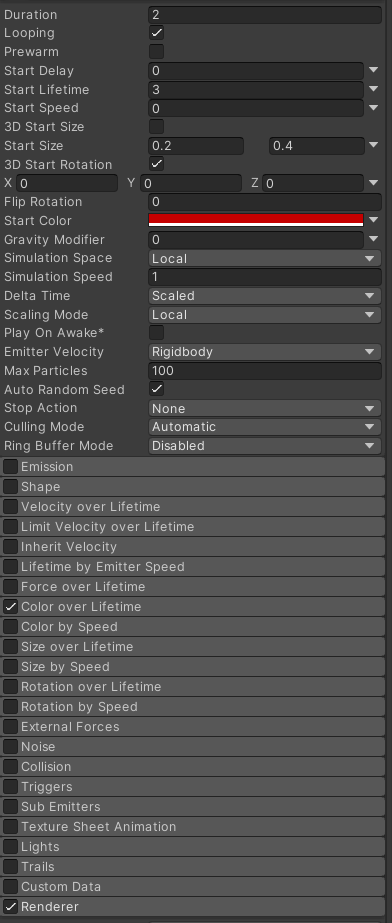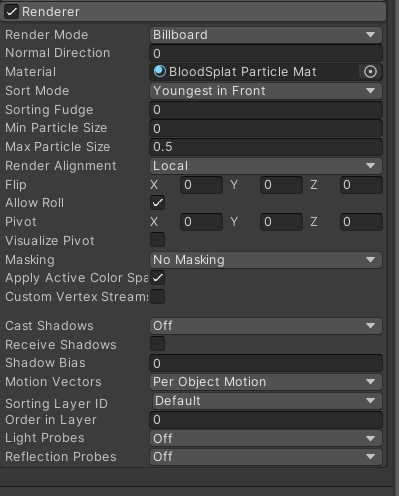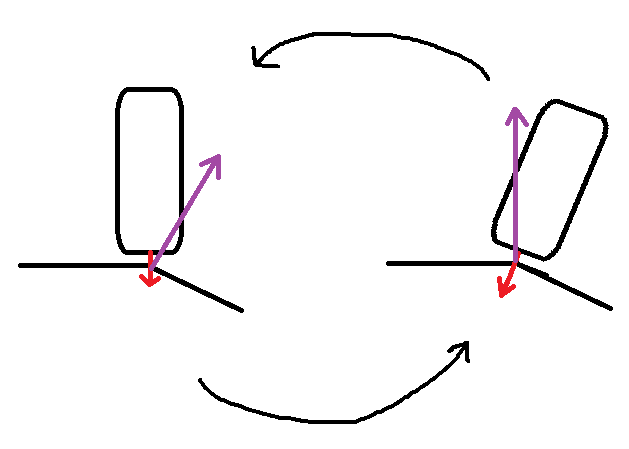## #StackBounty: #unity3d #rotation #particles #particle-system #decal Unity3D – Rotate Decal Particle from ParticleSystem to Match Normal

### Bounty: 50

I am trying to use a `ParticleSystem` for Decals as a cheap alternative but am unable to align the particles with all normal surfaces.

This is the nearest i’ve got that gets all axis correct except for an inverted `x` axis. (can see it’s the wrong way round on building windows)

``````if (Physics.Raycast(Camera.main.transform.position, Camera.main.transform.forward, out RaycastHit hit))
{
Quaternion rot = Quaternion.LookRotation(hit.normal);

ParticleSystem.EmitParams emitParams = new ParticleSystem.EmitParams
{
position = hit.point + 0.01f * hit.normal,
applyShapeToPosition = true,
rotation3D = rot.eulerAngles
};

_particleSystem.Emit(emitParams, 1);
}
``````

ParticleSystem SettingsParticleSystem Renderer SettingsI have tried multiple methods over the past 24 hours and have now got myself lost even though I’m sure its something simple, any help would be much appreciated.

Sorry about the image sizes scaling them down messed with the text

Edit: The editor and project settings are 2020.3.15F2 using URP

Get this bounty!!!

## #StackBounty: #unity #3d #rotation #raycasting Player rotation recalculation causes jittering on edges

### Bounty: 50

When my player walks around a circular planet everything works well, but when my planet is a shape like a cube or a dodecahedron, even with smoothed edges, my character jitters if I walk slowly on the edge.

That is because I raycast downward and set my characters rotation to be perpendicular with the normal. If im on the edge, the ray hits a different side of the shape than the one I stand on, causing my player to rotate to that new side, even if the angle between the face I stand on and the one I hit is 179°. So when My character rotates, the face I hit with the ray becomes the one I was standing on before, causing me to rotate back and forth between 2 faces.

Here is a beautiful scheme of what I mean:I tried using SphereCast instead of Raycast, but it didn’t work. How can I limit the back and forth rotations ?

Here is the script for the raycast:

``````void Update(){
``````

`````` if (Physics.Raycast(rayStart, GetDirection(IsGrounded, GravityChangeHitPoint), out RaycastHit hit, Mathf.Infinity, layerMask))
{
Debug.DrawLine(transform.position, hit.point,Color.red);
Quaternion matchSurface;

if (GravityChangeHitPoint == GravityCenter.position)
matchSurface = PlayerLookRotation(transform.forward, hit.normal);
else
matchSurface = PlayerLookRotation(transform.forward, -GetDirection(IsGrounded, GravityChangeHitPoint));

Quaternion cam = Quaternion.Euler(0, Input.GetAxis("Mouse X") * sensitivityX, 0);

if (!IsGrounded)
transform.rotation = Quaternion.Lerp(transform.rotation, matchSurface, Time.deltaTime * damping) * cam;
else
transform.rotation = matchSurface * cam;
}
}

Quaternion PlayerLookRotation(Vector3 approximateForward, Vector3 exactUp)
{
Quaternion zToUp = Quaternion.LookRotation(exactUp, -approximateForward);
Quaternion yToZ = Quaternion.Euler(90, 0, 0);

return zToUp * yToZ;
}
``````

Get this bounty!!!

## HackerRank: Circular Array Rotation

### Problem

John Watson performs an operation called a right circular rotation on an array of integers, `[a(0),a(1).a(2)...a(n-2),a(n-1)]`. After performing one right circular rotation operation, the array is transformed from

`[a(0),a(1).a(2)...a(n-2),a(n-1)]`

to

`[a(n-1),a(0),a(1).a(2)...a(n-2)]`.

Watson performs this operation `k` times. To test Sherlock’s ability to identify the current element at a particular position in the rotated array, Watson asks `q` queries, where each query consists of a single integer, `m`, for which you must print the element at index in the rotated array (i.e., the value of `a(m)`).

#### Input Format

The first line contains space-separated integers, `n`, `k`, and `q`, respectively.
The second line contains space-separated integers, where each integer `i` describes array element `a(i)`(where `0 <= i <= n`).
Each of the `q` subsequent lines contains a single integer denoting `m`.

#### Constraints

• `0 <= i <= 10^5`
• `0 <= a(i) <= 10^5`
• `0 <= k <= 10^5`
• `0 <= q <= 500`
• `0 <= m <= N-1`

#### Output Format

For each query, print the value of the element at index `m` of the rotated array on a new line.

```3 2 3
1 2 3
0
1
2
```
```2
3
1
```

#### Explanation

After the first rotation, the array becomes `[3,1,2]`.
After the second (and final) rotation, the array becomes `[2,3,1]`.

Let’s refer to the array’s final state as array `b`. For each query, we just have to print the value of `b(m)` on a new line:

• `m=0` , so we print `2` on a new line.
• `m=1` , so we print `3` on a new line.
• `m=2` , so we print `1` on a new line.